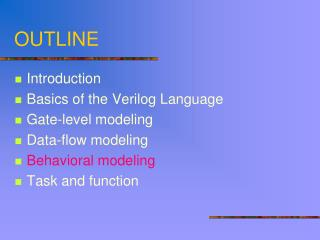Download PresentationOUTLINE# OUTLINE - PowerPoint PPT Presentation

Download Presentation##### OUTLINE

Download Policy: Content on the Website is provided to you AS IS for your information and personal use and may not be sold / licensed / shared on other websites without getting consent from its author. While downloading, if for some reason you are not able to download a presentation, the publisher may have deleted the file from their server.

- - - - - - - - - - - - - - - - - - - - - - - - - - - E N D - - - - - - - - - - - - - - - - - - - - - - - - - - -
##### Presentation Transcript

1. OUTLINE • Introduction • Basics of the Verilog Language • Gate-level modeling • Data-flow modeling • Behavioral modeling • Task and function

2. Behavioral modeling • Procedural blocks: • initial block: executes only once • always block:executes in a loop • Block execution is triggered based on user-specified conditions • always @ (posedge clk) ……… • All procedural blocks are automatically activated at time 0

3. Behavioral modeling • All procedural blocks are executed concurrently • reg is the main data type that is manipulated within a sequential block • It holds its value until assigned a new value

4. Initial Statement • Executes only once at the beginning of simulation initial statements • Multiple initial block execute concurrently at time 0 • Used for initialization and waveform generation reg [7:0] RAM[0:1023]; reg RIB_REG; initial begin integer INX; RIB_REG =0; for (INX = 0; INX < 1024; INX = INX + 1) RAM[INX] = 0; end group multiple statements

5. always Statement(1) • Executes continuously; must be used with some form of timing control always (timing_control) always statements CLK = ~CLK // Will loop indefinitely • Four forms of event expressions are often used • An OR of several identifiers (comb/seq logic) • The rising edge of a identifier (for clock signal of a register) • The falling edge of a identifier (for clock signal of a register) • Delay control (for waveform generator)

6. always Statement(2) • Any number of initial and always statements may appear within a module • Initial and always statements are all executed in parallel

7. Example module example (D, CURRENT_STATE, Q, NEXT_STATE); input D, CURRENT_STATE; output Q, NEXT_STATE; reg CLK, Q, NEXT_STATE; always #5 CLK = ~CLK; always @(posedge CLK) begin Q =D; end always @(negedge CLK) begin NEXT_STATE = CURRENT_STATE; end endmodule delay-controlled always block clock period = 10 activated when CLK has a 0 -> 1 transition activated when CLK has a 1 -> 0 transition

8. Procedural Assignments • The assignment statements that can be used inside an always or initial block • The target must be a register or integer type • always @(A or B) // infer wire begin B = A; C = B; end // C=A • always @(posedge CLK) // infer flip-flop begin B <= A; C <= B; D <= C; end

9. if and else if statements if (expression) statements { else if (expression) statements } [ else statements ] if (total < 60) begin grade = C; total_C = total_C + 1; end else if (sum < 75) begin grade = B; total_B = total_B + 1; end else grade = A; Conditional Statements

10. Conditional Statements • case statement case (case_expression) case_item_expression {, case_item_expression }: statements case_item_expression {, case_item_expression }: statements …… [ default: statements ] endcase case (OP_CODE) 2`b10: Z = A + B; 2`b11: Z = A – B; 2`b01: Z = A * B; 2`b00: Z = A / B; default: Z = 2`bx; endcase

11. Loop Statements • Four loop statements are supported • The for loop • The while loop • The repeat loop • The forever loop • The syntax of loop statements is very similar to that in C language • Most of the loop statements are not synthesizable in current commercial synthesizers

12. for loop • for (initial condition; terminating condition; increment) begin ….. end • for (i=0;i<32;i=i+1) state[i]=0; • for (i=0;i<32;i=i+2) begin state[i]=1; state[i+32]=0; end

13. repeat loop • repeat(constant number) • connot be used to loop on a general logical expression (a “while” loop is used for this purpose) • repeat(128) begin \$display(“count=%d”,count); count=count+1; end

14. forever loop • execute forever until the \$finish task is encountered. reg clock; initial begin clock = 1'b0; forever #5 clock = ~clock; // the clock flips every 5 time units. end initial #2000 \$finish;

15. while loop • while (logical expression) begin ……. end • while ((i<128) && continue) begin \$display(“count=%d”, count); i=i+1; end • while ((i<128) && continue) i=i+1;

16. Exercise

17. Homework-- ASM designed by HDL This example is referred from “Digital Design “, M. Morris Mano

18. Homework-- ASM designed by HDL

19. Homework-- ASM designed by HDL

20. Homework-- ASM designed by HDL

21. Homework-- ASM designed by HDL

22. Homework: Simulate and discuss the results